### Daniel J. Price, Christoph Federrath

[ Full text pdf (4.9Mb) ] accepted (8th April 2010) for publication in MNRAS (arXiv:1004.1446).

# Abstract

We compare the statistics of driven, supersonic turbulence at high Mach number using FLASH, a widely used Eulerian grid-based code and PHANTOM, a Lagrangian smoothed particle hydrodynamics (SPH) code at resolutions of up to 5123 in both grid cells and SPH particles. We find excellent agreement between codes on the basic statistical properties: a slope of k-1.95 in the velocity power spectrum for hydrodynamic, Mach 10 turbulence, evidence in both codes for a Kolmogorov-like slope of k-5/3 in the variable ρ1/3v as suggested by Kritsuk et al. (2007) and a log-normal PDF with a width that scales with Mach number and proportionality constant b=0.33-0.5 in the density variance--Mach number relation. The measured structure function slopes are not converged in either code at 5123 elements.

We find that, for measuring volumetric statistics such as the power spectrum slope and structure function scaling, SPH and grid codes give roughly comparable results when the number of SPH particles is approximately equal to the number of grid cells. In particular, to accurately measure the power spectrum slope in the inertial range, in the absence of sub-grid turbulence models, requires at least 5123 computational elements in either code. On the other hand the SPH code was found to be better at resolving dense structures, giving maximum densities at a resolution of 1283 particles that were similar to the maximum densities resolved in the grid code at 5123 cells, reflected also in the high density tail of the PDF. We find SPH to be more dissipative at comparable numbers of computational elements in statistics of the velocity field, but correspondingly less dissipative than the grid code in the statistics of density weighted quantities such as ρ1/3v.

For SPH simulations of high Mach number turbulence we find it important to use sufficient non-linear beta-viscosity in order to prevent particle interpenetration in shocks (we require βvisc = 4 instead of the widely used default value, βvisc = 2).

## MoviesMovies and images (c) 2010 Daniel Price and Christoph Federrath, released under a Creative Commons Attribution-Noncommercial-Share Alike 3.0 License.
[directory listing of all movies here]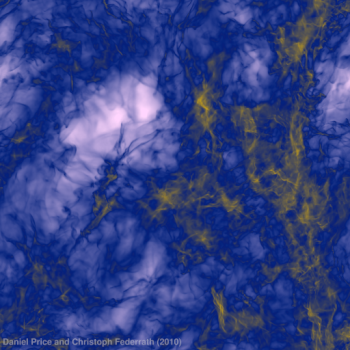Movie showing the projected column density in the PHANTOM (SPH) calculation using 134,217,728 particles (512 x 512 pixels, 10Mb Quicktime)

Full resolution version: (2046 x 2046 pixels, 47Mb Quicktime)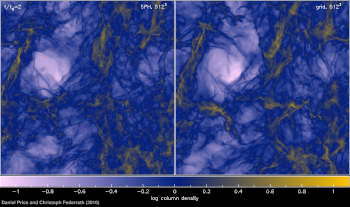Movie comparing the projected column density in the PHANTOM (SPH) calculations using 512^3 particles and FLASH (grid) calculations using 512^3 grid cells (11Mb Quicktime)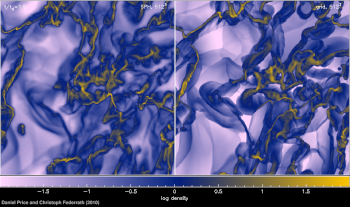Movie comparing cross-section slices of density at the midplane in the PHANTOM (SPH) calculations using 512^3 particles and FLASH (grid) calculations using 512^3 grid cells (10Mb Quicktime)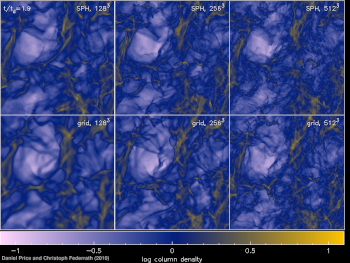Movie comparing the projected column density in the PHANTOM (SPH) calculations using 128^3, 256^3 and 512^3 particles (top row) and FLASH (grid) calculations using 128^3, 256^3 and 512^3 grid cells (bottom row) (12Mb Quicktime)

Full resolution version: (1537 x 1122 pixels, 23Mb Quicktime)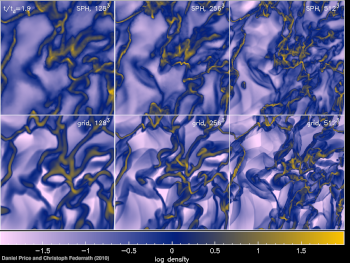Movie comparing cross-section slices of density at the midplane in the PHANTOM (SPH) calculations using 128^3, 256^3 and 512^3 particles (top row) and FLASH (grid) calculations using 128^3, 256^3 and 512^3 grid cells (bottom row) (12Mb Quicktime)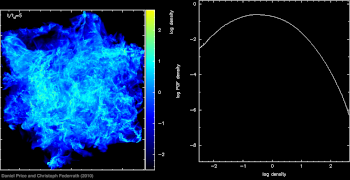Movie showing the development and time-variability of the log-normal Probability Distribution Function (right panel) in the PHANTOM calculation using 134,217,728 particles (7.3Mb Quicktime)

Images and movies were produced using SPLASH, a free visualisation tool for SPH data.